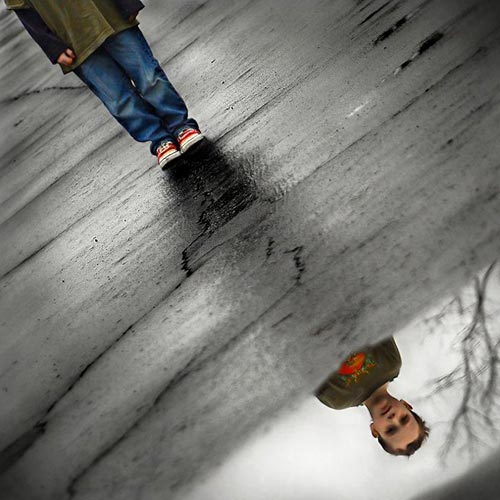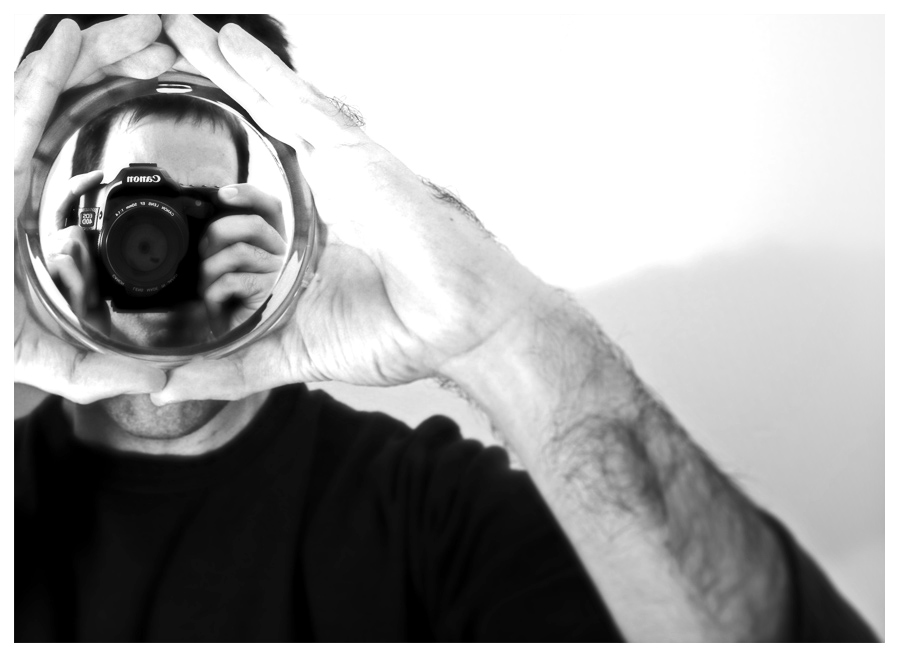# Portraits using the rule of thirds and the golden mean

Here are a few examples of some portraits I’ve found on the web that might inspire you for this week’s assignment…

diagonal rule of thirds:from here

golden mean:from here

golden mean:from here

vertical rule of thirds:from here

(arguably) rule of thirds (if you think the hair is a ‘third’):from here

rule of thirds where the focal point is on one of the intersections of the thirds:from here

golden mean:from here

rule of thirds where the focal point is on one of the main vertical lines:golden mean:from here